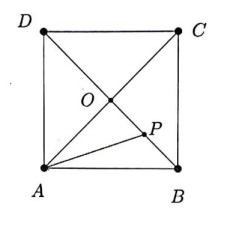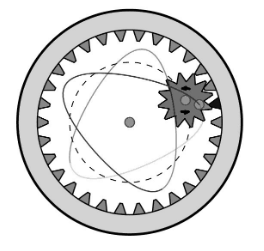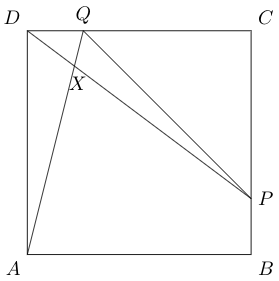# MT #11: Full-syllabus

### Instructions

• You are responsible for keeping time. Email all your solutions by 17:05 Hrs IST.
• Find an email from me with the subject line ‘Mock test 11: Full-syllabus’. Send your solutions (images) as replies to this email.
• Adjust/Reduce the resolution of the camera so that each image is less than 500 KB in size.
• Total marks: 100 (10x4=40 for Part A + 6x10=60 for Part B)
• As per the rules of CMI entrance exam, no calculators or log tables must be used.

For students who miss the live test (members only)
Self-administer the mock test and email your solutions before 10 July, 23:59 Hrs. Your solutions will be evaluated but marks won’t be counted for official use in the future. Solutions submitted after 10 July, 23:59 Hrs will not be evaluated.

## Part A: Short-answer type questions

Submission file: Write answers to all the ten questions on a single sheet of paper. Email a picture of your answer sheet. Name the file as PartA.jpg or PartA.png.

For this part, answers must be written without any explanation.

1. $$ABCD$$ is a unit square and $$P$$ is the midpoint of segment $$OB$$ in the figure below. What is the length of $$AP$$?2. Suppose $$2 \log x+\log y=x-y$$. Then the equation of the tangent line to the graph of this equation at the point $$(1,1)$$ is:
(a) $$x+2 y=3$$
(b) $$x-2 y=3$$
(c) $$2 x+y=3$$
(d) $$2 x-y=3$$
3. How many triples $$({A}, {B}, {C})$$ are there of sets with union $${A} \cup {B} \cup {C}=\{1,2,3,4,5,6,7,8\}$$ and $${A} \cap {B} \cap {C}=\varnothing ?$$

4. Find all integers $$x$$ for which $$|x^3+2x|$$ is prime.

5. Given that $$a_{1}=2, a_{2}=3, a_{n}=a_{n-1}+2 a_{n-2}$$, what is $$a_{19}+a_{20}$$?

6. A spirograph is a toy that has a cog and a rack. The cog and the rack have teeth that fit together. In the figure shown below, the cog has 12 teeth and the rack has 30.The cog has a hole near its circumference. If we put a pen in the hole and turn the cog around the rack many times, we get a pattern. In the example shown above, we get a pattern with five pointed ends. Pointed ends are those points in the pattern where the pen is closest to the rack.

Suppose the cog had 9 teeth and the rack had 20. How may pointed ends would the pattern have?

7. $\lim_{x \rightarrow 0}\left(\frac{\sin x}{x}\right)^{\frac{1}{1-\cos x}}$

8. How many positive integers divide at least one of $$10^{30}$$ and $$20^{30}$$ ?

9. Suppose that the set $$\{1,2, \cdots, 2022\}$$ has been partitioned into disjoint pairs $$\left\{a_{i}, b_{i}\right\}(1 \leq i \leq 1011)$$ so that for all $$i,\left|a_{i}-b_{i}\right|$$ equals 1 or 6. Find all the possible values for the last digit of the sum: $\left|a_{1}-b_{1}\right|+\left|a_{2}-b_{2}\right|+\cdots+\left|a_{1011}-b_{1011}\right|$

10. There is a highway on which a group of police officers are testing for drunk-driving. They have breathalyzers that raises a false alarm in 5% of the cases in which the driver is sober. That is, even if the driver is not drunk there is a 5% chance that the breathalyzer incorrectly shows him to be drunk. However, the breathalyzer never fails to detect a truly drunk person. We know for a fact that one in a every thousand drivers on the highway is driving drunk. Suppose the police officers stop a driver at random to administer a breathalyzer test. It indicates that the driver is drunk. The probability that he is truly drunk is around:
(a) 2%
(b) 10%
(c) 20%
(d) 95%

## Part B: Subjective questions

Submission files: Each question in this part must be answered on a page of its own. Name the files as B1.jpg, B2.jpg, etc. In case you have multiple files for the same question, say B4, name the corresponding files as B4-1.jpg, B4-2.jpg, etc.

Clearly explain your entire reasoning. No credit will be given without reasoning. Partial solutions may get partial credit.

B1. Prove or disprove: There are two numbers $$x, y$$ such that: \begin{align*} x+y&=1\\ x^{2}+y^{2}&=2\\ x^{3}+y^{3}&=3 \end{align*}

B2. In the figure shown below, $$ABCD$$ is a square with side length of $$4$$ cm. $$DQ=BP=1$$ cm. Line segments $$AQ$$ and $$DP$$ intersect at point $$X$$. Find the area of the triangle $$PQX$$.B3. (a) Suppose we have a polynomial $$p(x) = a_n x^n + a_{n-1}x^{n-1} + \cdots + a_0$$ where $$a_i$$s are real numbers. If $$\sum \frac{a_i}{i+1} = 0$$, prove that $$p(x)$$ has a real root between 0 and 1. $$\;\;\;$$ [4 marks]
(b) Evaluate the following integral: $\int_{0}^{\pi} \frac{x \sin x}{1+\cos ^{2} x} d x \;\;\;\;\;\;\;\; \text{[6 marks]}$

B4. Determine all polynomials $$f(x)=a x^{2}+b x+c$$ such that $$f(a)=a, f(b)=b$$ and $$f(c)=c$$.

B5. A special matrix is a square matrix whose entries are $$0$$ or $$1$$ such that, in each row, the $$1$$s appear as consecutive entries. For example, the following is a $$4 \times 4$$ special matrix. $\left[\begin{array}{llll} 0 & 1 & 1 & 1 \\ 0 & 1 & 1 & 0 \\ 0 & 1 & 1 & 0 \\ 1 & 1 & 1 & 1 \end{array}\right]$ Show that the determinant of any special matrix is either $$1,-1$$ or $$0$$.

B6. Show that the number $$4^{2020}$$ cannot be written as a sum of three cubes. In other words, prove that there are no integers $$a_1,a_2$$ and $$a_3$$ such that $a_1^3 + a_2^3 + a_3^3 = 4^{2020}$